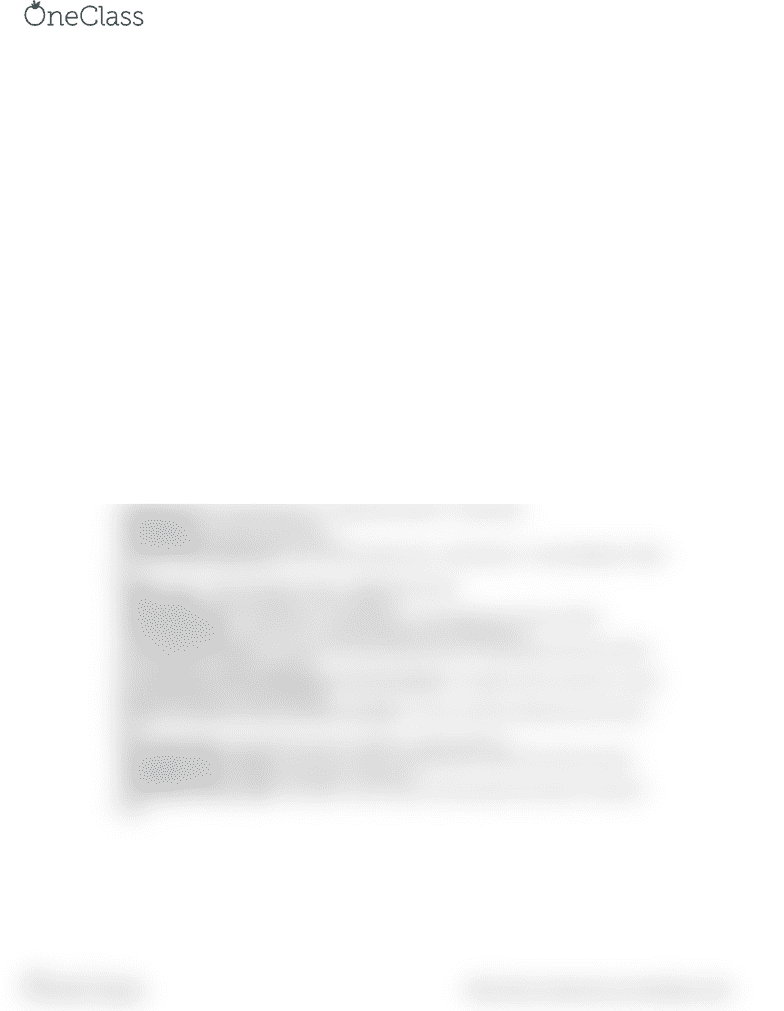# International Business Administration SIB500 Study Guide - Final Guide: Contribution Margin, Earnings Before Interest And Taxes, Asset Turnover

42 views1 pagesSIB500 Formulas
- Department overhead rate = Manufacturing overhead / machine hours
- Total costs = f + xv (f = total fixed costs, x = volume of activity, v = variable cost per unit of activity)
- Prime Costs = Direct materials + Direct Labour
- Conversion Costs = Manufacturing overhead + Direct labour
- Predetermined MOH rate = Total estimated manufacturing overhead costs / total estimated amount of
the allocation base
- Cost of Goods Sold = Number of units * Manufacturing cost per unit
- Total Mixed Costs (y) = Variable costs (vx) + Fixed cost (f)
- Operating Leverage Factor = Contribution Margin / Operating Income
- Unit Contribution Margin = Sales price per unit – Variable cost per unit
- Total Contribution Margin = Sales revenue * contribution margin ratio
- Operating income = Sales revenue * contribution margin ratio – fixed costs ---OR--- CM – Fixed expenses
- Contribution Margin Ratio = Contribution margin / sales revenue
- Direct Materials Price Variance: (AP-SP)*AQ OR Total AP – Total SP
- Direct Materials Efficiency Variance: (AQ-SQ)*SP
- Direct Labour Price Variance: (AP-SP)*AQ
- Direct Labour Efficiency Variance: (AQ-SQ)*SP
- U = Unfavorable, F = Favorable
- Total Variance = Price + Efficiency
- ROI (%) = Operating Income / Total Assets ---OR--- Profit margin * Asset turnover
- Sales = Operating income / Sales margin percentage
- Profit/Sales Margin (%) = Operating Income / Sales
- Capital/Asset Turnover = Sales / Total Assets
- Capital Charge = Operating assets * Cost of capital
- Residual Income = Operating Income – (Target Rate of Return * Total Assets)
- Profit Margin = Net income / Net sales
- Operating Assets = Sales / Asset Turnover
- Economic Value Added (EVA) = After-Tax Operating Income – [(Total Assets – Current liabilities) * WACC
%)]
- Budget = Sales + Desired Ending Inventory – Beginning Inventory
- Cash Available = Beg cash Balance + Cash Collections
- Ending Cash Balance = Cash available – cash payments + Financing (borrow, repay, interest)
- Annual Depreciation = (Initial Cost – Estimated Selling Value) / Estimated Life
- Accounting Rate of Return = Average Annual Operating Inventory (Or Operating income from asset) /
Initial Inventory (or initial investment)
- Accounting Rate of Return (Depreciation is the only expense) = Average annual net cash flow – annual
depreciation expense / initial investment
- Payback Period (when annual cash flows are EQUAL) = Amount invested / Expected annual net cash
flow
- Net Present Value = PV of all future returns combined – initial investment
- Annual Depreciation Expense = Initial cost of asset – Residual value / Useful life of asset (in years)
- Profitability Index = PV of net cash inflows / Investment
- Internal Rate of Return (IRR) = Investment’s cost / Amount of each equal net cash flow = Annuity PV
Factor
find more resources at oneclass.com
find more resources at oneclass.com
Unlock document

This preview shows half of the first page of the document.
Unlock all 1 pages and 3 million more documents.

Already have an account? Log in

# Get access

Grade+
\$10 USD/m
Billed \$120 USD annually
Homework Help
Class Notes
Textbook Notes
40 Verified Answers
Study Guides
1 Booster Class